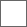### 外交术的研究

1、降低投降费用，每一级降低20%，满级降低60%投降费用。

2、减少进入图书馆的门槛，每一级降低2级，满级外交的话，4级英雄就可以进入图书馆获得加成。

3、使野怪更容易加入，具体在下面楼层分析（图片的加入几率是不准确的）。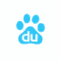（0/1/2）：英雄身上有和外交兵种一致的兵种时，取值2； 英雄身上有和外交兵种同一种族的兵种时，取值1； 其他情况取值0。1、屈服的，最先进行判断，只要野怪敌意值为0，则必定加入（无视其他条件）。
2、野蛮的（敌意10），只有打一架和逃跑两种情况，不会加入。
3、中间三个敌意区间，有打一架、逃跑、仰慕和金钱加入四种情况。

1、先判定野怪敌意是否为0，为0则直接加入，不为0进入下一步

2、对比英雄军队实力（兵力、属性）和野怪实力，得到打一架或者逃跑两种情况。当英雄军队实力远超野怪时，野怪会逃跑，进入第3步的判断。
PS：野怪敌意越高，己方军队实力需要高出野怪越多，才会出现逃跑的情况。

3、会逃跑的野怪，将其敌意值和英雄的仰慕外交度相比较，当仰慕外交度大于等于敌意值时，野怪仰慕（免费加入），当仰慕外交度小于敌意值时，进入第4步

4、将野怪敌意值和英雄的金钱外交度相比较，当金钱外交度大于等于敌意值时，野怪收费加入，否则逃跑2020-08-07 19:33 广告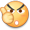研究贴……尽管我不用外交术，也要支持一下……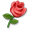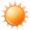Diplomacy internals (for HoMM III 3.2 and 4.0)

1. [Hero_Power]
Hero_Power = sqrt((1 + 0.05 * Attack) * (1 + 0.05 * Defense));

2. [Total_Army_Power]
Total_Army_Power = floor(Hero_Power * Army_Power);

3. [k]
k = Total_Army_Power / Neutrals_Power;

Note: neutrals number cannot exceed 4000. Have you ever seen more?

4. [Power_Factor] (fixed)
Power_Factor = 11 if k >= 7;
Power_Factor = floor(2*(k - 1)) if 1 <= k < 7;
Power_Factor = -1 if 0.5 < k < 1;
Power_Factor = -2 if 0.333 < k <= 0.5;
Power_Factor = -3 if k <= 0.333;

Note: 0.333 means 333/1000, not 1/3!
Now I am sure￼

5. [Sympathy]
Sympathy = 0 if there are no creatures of the same kind as neutrals in hero's army;
Sympathy = 1 if there is at least one creature (or upgrade/downgrade) of the same kind as neutrals in hero's army;
Sympathy = 2 if total number of creatures (or their upgrades/downgrades) of the same kind as neutrals in hero's army are strictly bigger than number of all the other beings in the army;

The notes below are for join calculations only!
Note: earth, water and fire elementals don't have upgrades while air ones do!
Note: magma, ice and energy elementals don't have downgrades while storm ones do!

6. [Charisma (Ch)]
Ch = Power_Factor + Diplomacy_Level + Sympathy;

7. [Aggression (X)]
X = -4 (always) for compliant neutrals;
X = 10 (always) for savage neutrals;
X = 1..7 for friendly neutrals;
X = 1..10! for aggressive neutrals;
X = 4..10! for hostile neutrals;

Note: for friendly, aggressive and hostile neutrals Õ is a pseudo-random value which is generated at creation of new game. You can see it when using LM Oracle 3.72 program.

8. if Ch < X neutrals will fight;

9. if Ch >= X there are two cases:
b)Only if the first condition is false:ifSympathy + 2 * Diplomacy_Level + 1 >= Xneutrals willjoin for goldprovided you have enough money to buy them all (no resources are needed).

Note: if you reject an offer to join monst

Note: if you reject an offer to join monsters:
a) flee (if Ch > X and they CAN flee i.e. they are not set to never flee);
b) take offence and attack (if Ch = X).

Note:on Easy difficultall heroes have a hidden Diplomacy level which is used for join calculations (only?). For example, if your hero has Basic Diplomacy then Diplomacy_Level istwoinstead ofone. Nevertheless, Diplomacy_Level cannot exceedthree

-----
2Rainalcar:
In your case Ch = 0 + 3 + 2 = 5 (Power_Factor = 0, Diplomacy_Level = 3, Sympathy = 2).

Sympathy + Diplomacy_Level + 1 = 2 + 3 + 1 = 6.
Sympathy + 2 * Diplomacy_Level + 1 = 2 + 6 + 1 = 9.

So, you couldn't join neutrals with X > 5 (they fight you).
if X <= 5 AA'salways join for free because 6 >= X is true in this case.

There are the next cases for hostile neutrals:
a) X = 6, 7, 8, 9, 10 out of X = 4, 5, 6, 7, 8, 9, 10 => fight (in your case)/flee probability is 5/7 = 71%;
b) X = 4, 5 out of X = 4, 5, 6, 7, 8, 9, 10 => free join probability is 2/7 = 29%.

Now try to make the same test with 20 AA's of yours vs 10 AA's!
Power_Factor = 2, Ch = 2 + 3 + 2 = 7.
In this case you will never join neutrals with X > 7.
If X = 7, for example, you will never join them for free as well (6 >= 7 is false) but you easily join them for gold (9 >= 7 is true)!

For hostile neutrals in this case:
a) X = 8, 9, 10 out of X = 4..10 =>fight/flee probability is 3/7 = 43%;
b) X = 7 out of X = 4..10 => money join probability is 1/7 = 14%;
c) X = 4, 5, 6 out of X = 4..10 => free join probability is 3/7 = 43%.